Previous Page | Next Page

 Module Library

## RANDMVT Function

generates a random sample from a multivariate Student's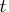distribution

RANDMVT( N, DF, Mean, Cov )

The inputs are as follows: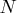is the number of desired observations sampled from the multivariate Student'sdistribution.

DF
is a scalar value representing the degrees of freedom for thedistribution.
Mean
is a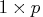vector of means.
Cov
is a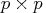symmetric positive definite variance-covariance matrix.

The RANDMVT function returns an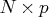matrix containingrandom draws from the Student'sdistribution with DF degrees of freedom, mean vector Mean, and covariance matrix Cov.

If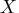follows a multivariatedistribution with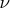degrees of freedom, mean vector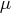, and variance-covariance matrix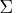, then
• the probability density function for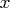is
• if
, the probability density function reduces to a univariate Student'sdistribution.
• the expected value of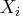is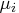.
• the covariance ofand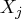is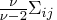when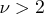.

The following example generates 1000 samples from a two-dimensionaldistribution with 7 degrees of freedom, mean vector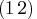, and covariance matrix S. Each row of the returned matrix x is a row vector sampled from thedistribution. The example then computes the sample mean and covariance and compares them with the expected values. Here are the code and the output:


call randseed(1);
N=1000;
DF = 4;
Mean = {1 2};
S = {1 1, 1 5};
x = RandMVT( N, DF, Mean, S );
SampleMean = x[:,];
n = nrow(x);
y = x - repeat( SampleMean, n );
SampleCov = y*y / (n-1);
Cov = (DF/(DF-2)) * S;
print SampleMean Mean, SampleCov Cov;

SampleMean                Mean

1.0768636 2.0893911         1         2

SampleCov                 Cov

1.8067811 1.8413406         2         2
1.8413406 9.7900638         2        10


In the preceding example, the columns (marginals) of x do not follow univariatedistributions. If you want a sample whose marginals are univariate, then you need to scale each column of the output matrix:


x = RandMVT( N, DF, Mean, S );
StdX = x / sqrt(diag(S)); /* StdX columns are univariate t */
`

Equivalently, you can generate samples whose marginals are univariateby passing in a correlation matrix instead of a general covariance matrix.

For further details about sampling from the multivariatedistribution, see Kotz and Nadarajah (2004, pp. 1 - 11).Previous Page | Next Page | Top of Page